Courses

# Limits of Trigonometric Functions JEE Notes | EduRev

## JEE : Limits of Trigonometric Functions JEE Notes | EduRev

The document Limits of Trigonometric Functions JEE Notes | EduRev is a part of the JEE Course Mathematics (Maths) Class 11.
All you need of JEE at this link: JEE

limit of Trigonometric Functions

(a)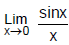= 1 (approach from left side)

(b)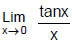= 1 (approach from right side)

(c)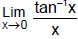= 1

(d)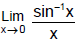= 1

[Where x is measured in radians]

Ex.17 Find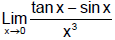Sol.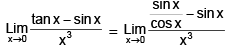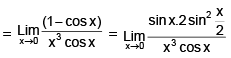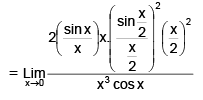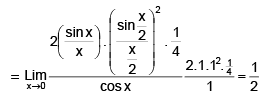Ex.18 Evaluate :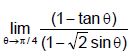Sol.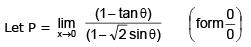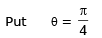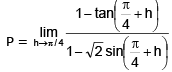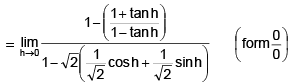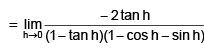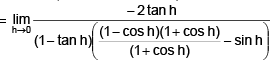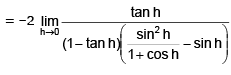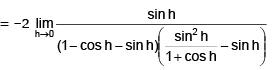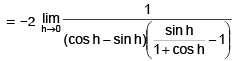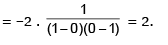Ex.19 Solve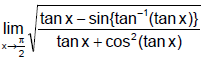Sol.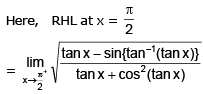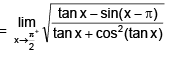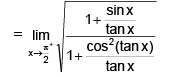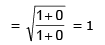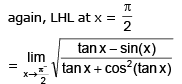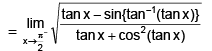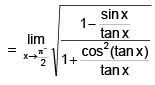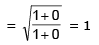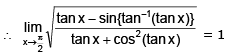Note : Limit Using Expansion of Functions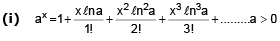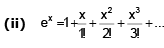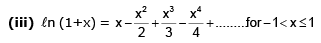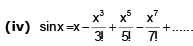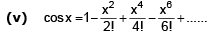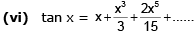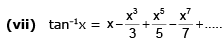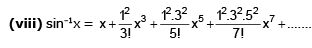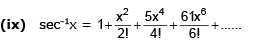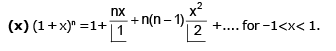Ex.20 Find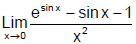Sol.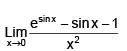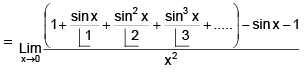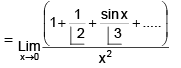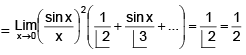Ex.21 Find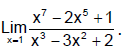Sol.

This is of the form 0/0 if we put x = 1. Therefore we put x = 1 + h and expand.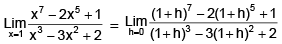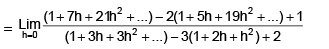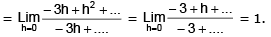Ex.22 Evaluate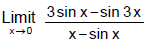Sol.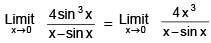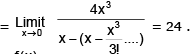Ex.23 Let f(x) be a function such that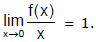Find the values of a and b such that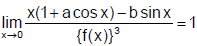Sol.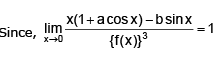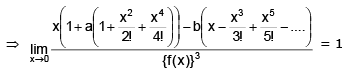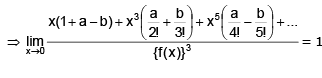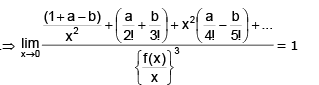R.H.S. is finite then L.H.S. is also finite, then 1 + a –b = 0  and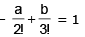Ex.24 If the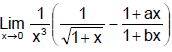exists and has the value equal to l, then find the value of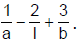Sol.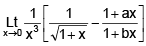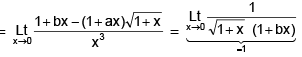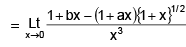Use binomial expansion to get the following relations :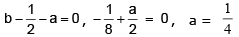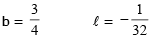Offer running on EduRev: Apply code STAYHOME200 to get INR 200 off on our premium plan EduRev Infinity!

## Mathematics (Maths) Class 11

158 videos|186 docs|161 tests

,

,

,

,

,

,

,

,

,

,

,

,

,

,

,

,

,

,

,

,

,

;